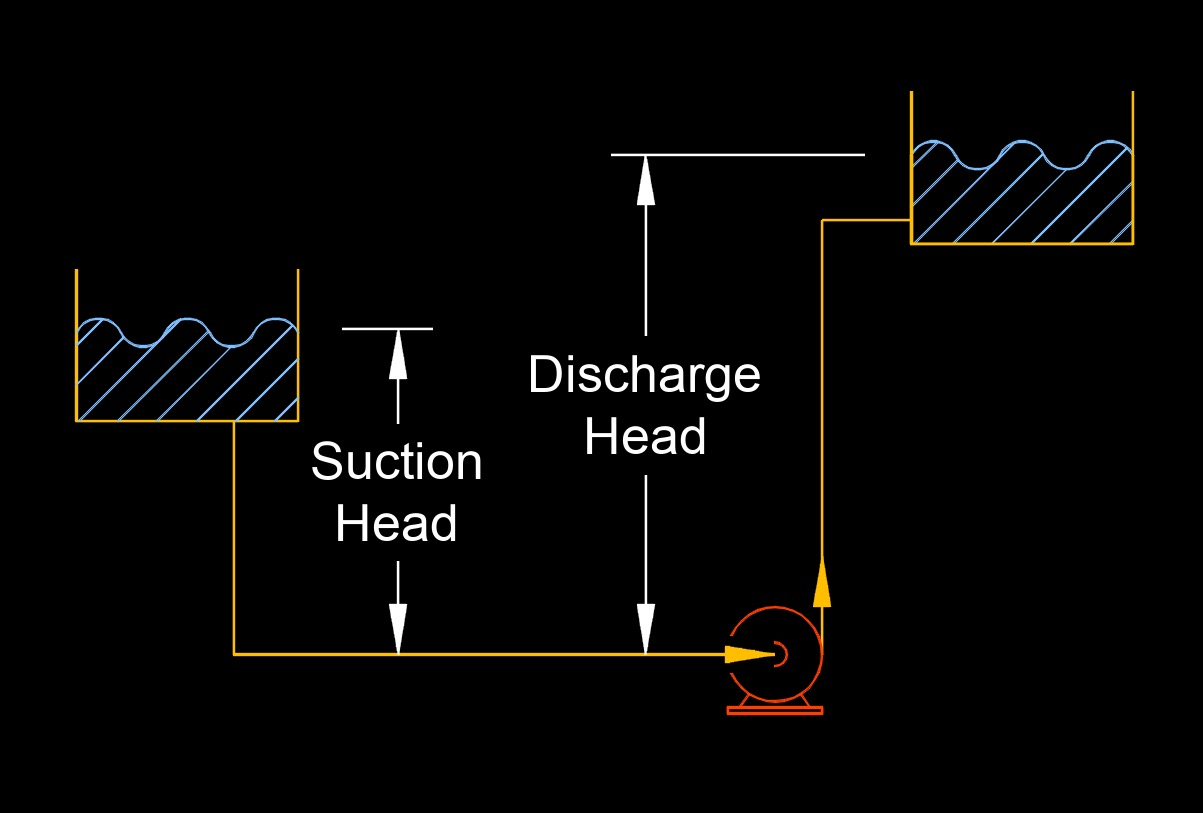on . Posted in Fluid DynamicsSystem head is the difference between the total discharge head minus the total suction head.

$$\large{ H = h_d - h_s }$$     (System Head)

$$\large{ h_d = H + h_s }$$

$$\large{ h_s = h_d - H }$$

Symbol English Metric
$$\large{ H }$$ = system head $$\large{ft}$$  $$\large{m}$$
$$\large{ h_d }$$ = total discharge head $$\large{ft}$$ $$\large{m}$$
$$\large{ h_s }$$ = total suction head $$\large{ft}$$ $$\large{m}$$